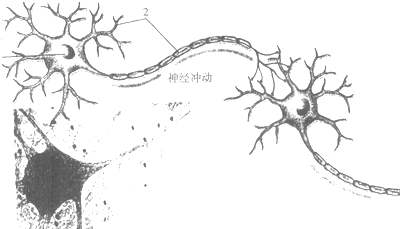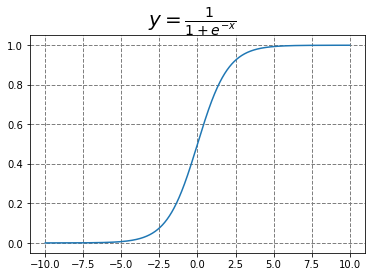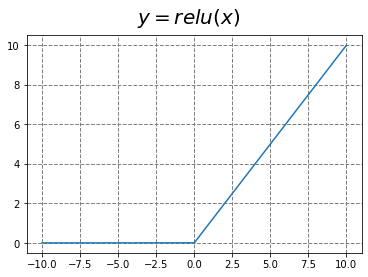# [column 2] activation function (1) on activation function and its development

Time：2020-11-27

## [column 2] activation function (1) on activation function and its development

Activation function is a very important part of neural networks. In the history of neural networks, various activation functions are also a research direction. In our study, we often don’t think about why we use this function and where they come from?Biological neural network has given a lot of inspiration to artificial neural network. As shown in the figure above, the signals from dendrites accumulate continuously. If the signal strength exceeds a certain threshold, the signals continue to transmit to the axons. If not, the signal is “killed” by neurons and cannot continue to spread.

In the artificial neural network, activation function has the same effect. Imagine that when we learn something new, some neurons will produce different output signals, which makes the neurons connect.

Sigmoid function may be the first activation function that you are exposed to when you are learning neural network. We know that it has many good characteristics, such as the ability to transform continuous real values into 0 to 1 output, and the derivation is simple. Then how to get this function? This paper provides an angle from the maximum entropy principle.

### 1 sigmoid function and softmax function

#### 1.1 maximum entropy principle and model

The maximum entropy principle is a criterion for probabilistic model learning. According to the principle of maximum entropy, among all possible probability models, the model with the largest entropy is the best one.

Assuming discrete random variablesThe probability distribution of isThen its entropy is zeroEntropy satisfies the following inequality:Where,yesIf and only ifThe equal sign on the right holds when the distribution of is uniform. That is to say, whenThe entropy is maximum when the distribution is uniform.

Intuitively speaking, this principle holds that the probability model to be selected must first satisfy the existing conditions. Without more information, no other uncertain parts are equally possible.

The probability distribution model is a conditional distribution modelGiven a training setIt can be determinedEmpirical distribution and marginal distributionThe empirical distributions were as followsandexpress.

Feature functionDescription inputandBetween a fact, defined as:From the above information, it can be assumed thatOn empirical distributionThe expected value of and about the modelAnd empirical distributionThe expected values of are equalCombined with the conditions, the problem is equivalent to the constrained optimization problemBy using Lagrange multiplier method, the problem is transformed into finding the minimum value of the following formulaAt this point, we are rightseekDerivative of:Let the derivative be 0, and theIn this case, the solution is as follows:becauseThe results are as follows:From the above two formulas, we can get:It is not difficult for careful students to find that this is very similar to the softmax functionThe softmax function is obtainedWhat about the sigmoid function? In fact, this function is a special case of softmaxHaving finished the derivation, we will talk about the characteristics of these two functions. The advantages of sigmoid function have been mentioned before, but sigmoid is prone to the phenomenon of “gradient disappear” in the back propagation.It can be seen that when the input value is large or small, its derivative is close to 0, which will lead to too small gradient to train.

### The rise of relu function familyAs shown in the figure, the RLU function can avoid the problem of gradient disappearance. Compared with sigmoid / tanh function, the advantages of relu activation function are as follows:

• When the gradient descent (GD) method is used, the convergence rate is faster.
• Compared with relu, only one threshold value is needed to get the activation value, and the calculation speed is faster.

The disadvantage is: when the input value of relu is negative, the output is always 0, and its first derivative is always 0, which will lead to the neuron unable to update the parameters, that is, the neuron will not learn. This phenomenon is called “dead neuron”.

In order to solve the problem of relu function, there are many development based on relu function, such as leaky relu (relu with leakage unit), rrelu (random relu), and so on. Maybe you can find a better relu function one day!

### quote

 [Li Hang. Statistical learning methods [M]. Tsinghua University Press, 2012

## 15. How to rank the scores of millions of candidates

How to rank the scores of millions of candidates Focus on “code brother byte”. Here are algorithm series, big data storage series, spring series, source code architecture disassembly series, interview series Coming soon. Don’t get lost by setting star In fact, counting sort is a special case of bucket sort. Bucket sortingThe core idea is […]